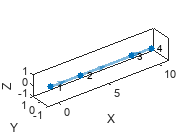# plot

Plot view set views and connections

## Syntax

``plot(vSet)``
``h = plot(vSet)``
``plot(vSet,Name,Value)``

## Description

````plot(vSet)` plots the views and connections in the view set, `vSet`.```
````h = plot(vSet)` returns a graph plot, `h````
````plot(vSet,Name,Value)` specifies options using one or more name-value pair arguments.```

## Examples

collapse all

Create an empty image view set.

`vSet = imageviewset;`

Define three relative poses.

```relPoses = repelem(rigid3d,3,1); relPoses(1).Translation = [3 0 0]; relPoses(2).Translation = [5 0 0]; relPoses(3).Translation = [2 0 0];```

```absPoses = repelem(rigid3d,4,1); absPoses(2).T = relPoses(1).T*absPoses(1).T; absPoses(3).T = relPoses(2).T*absPoses(2).T; absPoses(4).T = relPoses(3).T*absPoses(3).T;```

Add four views to the image view set.

```vSet = addView(vSet,1,absPoses(1)); vSet = addView(vSet,2,absPoses(2)); vSet = addView(vSet,3,absPoses(3)); vSet = addView(vSet,4,absPoses(4));```

```vSet = addConnection(vSet,1,2,relPoses(1)); vSet = addConnection(vSet,2,3,relPoses(2)); vSet = addConnection(vSet,3,4,relPoses(3));```

Plot the view set with view identifers.

`plot(vSet,'ShowViewIds','on')`## Input Arguments

collapse all

Image view set, specified as a `imageviewset` object.

### Name-Value Arguments

Specify optional comma-separated pairs of `Name,Value` arguments. `Name` is the argument name and `Value` is the corresponding value. `Name` must appear inside quotes. You can specify several name and value pair arguments in any order as `Name1,Value1,...,NameN,ValueN`.

Example: `'ShowViewIds',off`

Axes on which to plot view set, specified as the comma-separated pair consisting of '`Parent`' and an `Axes` object. To create an `Axes` object, use the `axes` function.

Display of view identifiers, specified as the comma-separated pair consisting of `'ShowViewIds'` and `'on'` or `'off'`.

## Output Arguments

collapse all

Graph plot, returned as a `GraphPlot` object. You can use the `GraphPlot` object functions and properties to inspect and adjust the plotted graph.

### Objects

Introduced in R2020a